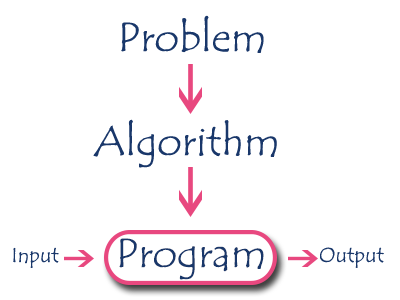The perfect place for easy learning...

×

# What is an algorithm?

An algorithm is a step by step procedure to solve a problem. In normal language, the algorithm is defined as a sequence of statements which are used to perform a task. In computer science, an algorithm can be defined as follows...

An algorithm is a sequence of unambiguous instructions used for solving a problem, which can be implemented (as a program) on a computer.

Algorithms are used to convert our problem solution into step by step statements. These statements can be converted into computer programming instructions which form a program. This program is executed by a computer to produce a solution. Here, the program takes required data as input, processes data according to the program instructions and finally produces a result as shown in the following picture.# Specifications of Algorithms

Every algorithm must satisfy the following specifications...

1. Input - Every algorithm must take zero or more number of input values from external.
2. Output - Every algorithm must produce an output as result.
3. Definiteness - Every statement/instruction in an algorithm must be clear and unambiguous (only one interpretation).
4. Finiteness - For all different cases, the algorithm must produce result within a finite number of steps.
5. Effectiveness - Every instruction must be basic enough to be carried out and it also must be feasible.

# Example for an Algorithm

Let us consider the following problem for finding the largest value in a given list of values.
Problem Statement : Find the largest number in the given list of numbers?
Input : A list of positive integer numbers. (List must contain at least one number).
Output : The largest number in the given list of positive integer numbers.

Consider the given list of numbers as 'L' (input), and the largest number as 'max' (Output).

# Algorithm

1. Step 1: Define a variable 'max' and initialize with '0'.
2. Step 2: Compare first number (say 'x') in the list 'L' with 'max', if 'x' is larger than 'max', set 'max' to 'x'.
3. Step 3: Repeat step 2 for all numbers in the list 'L'.
4. Step 4: Display the value of 'max' as a result.

# Code using C Programming Language

``````int findMax(L)
{
int max = 0,i;
for(i=0; i < listSize; i++)
{
if(L[i] > max)
max = L[i];
}
return max;
}```
```

# Recursive Algorithm

In computer science, all algorithms are implemented with programming language functions. We can view a function as something that is invoked (called) by another function. It executes its code and then returns control to the calling function. Here, a function can be called by itself or it may call another function which in turn call the same function inside it is known as recursion. A recursive function can be defined as follows...

The function which is called by itself is known as Direct Recursive function (or Recursive function)

A recursive algorithm can also be defined as follows...

The function which calls a function and that function calls its called function is known Indirect Recursive function (or Recursive function)

Most of the computer science students think that recursive is a technique useful for only a few special problems like computing factorials, Ackermann's function, etc., This is unfortunate because the function implemented using assignment or if-else or while or looping statements can also be implemented using recursive functions. This recursive function is very easier to understand when compared to its iterative counterpart.